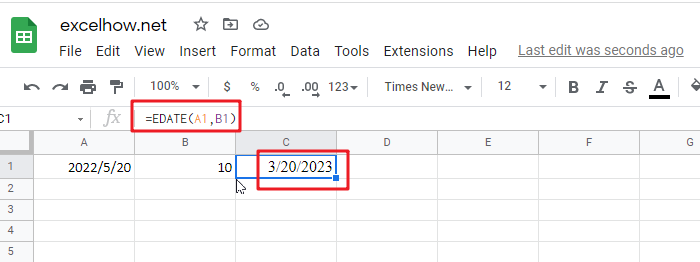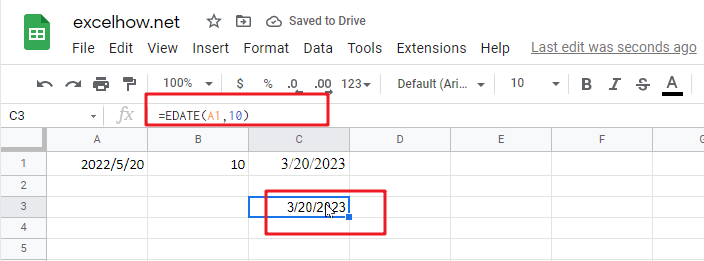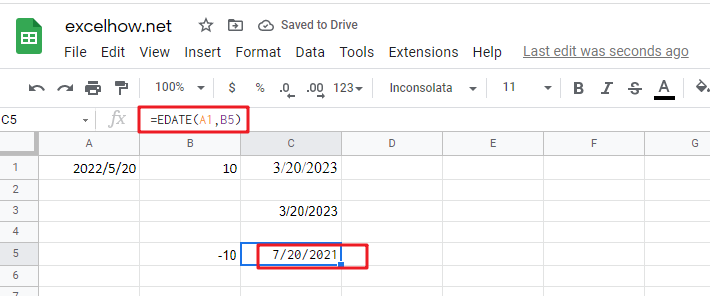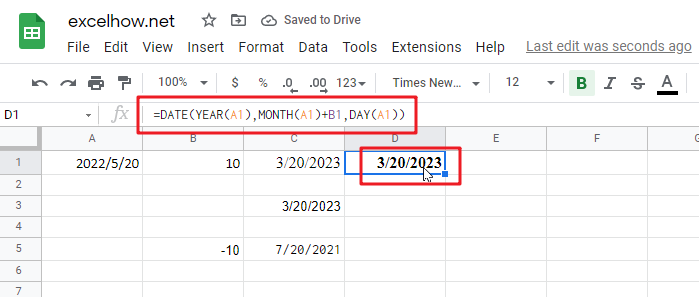# ExcelHow

It is important to adjust time periods when performing financial modeling. In Google Sheets, you can use the MONTH function to add a specific number of months to a date. The MONTH function takes one argument: the number of months you want to add. Here will show you how to add months to date in Google Sheets and even provide some examples that might be useful!## Method1: Add Months to Date using EDATE Function

Generic formula:

`=EDATE(Sart_date, months)`

### Arguments

• `Start_date`: the starting date on which you want to add months
• `Months`: the month that you wish to add

### Return Value:

The function will return the date that is the indicated number of months after the start_date. If you enter a negative number of months, it’ll count back from the end date.

When you enter the MONTH function, Google Sheets looks at the date in the cell that you specify as the start_date argument. It then adds the number of months that you entered as the second argument to that date. If you enter a negative number for the second argument, Google Sheets subtracts that number of months from the start_date.

To add a number of months is placed at B2 and add months to the date in cell A2. The formula for this would be:

`=EDATE(A1,B1)`Or simply enter the number you want in your formula, and it will be replaced with that value.

`=EDATE(A1,10)`### Explanation

This will add 10 months to the date in cell A1. The function will return the date that is the indicated number of months after the start_date. If you enter a negative number of months, it’ll count back from the end date.

The EDATE function is a great way to find out your next date. Simply enter any valid date and then select how many months away from now you want the new one! It’s really easy; just feed it with an amount less than or equal to the number of desired days between these two points-and voila.## Method2: Add Months to Date using Date Function

Generic formula:

`=DATE(YEAR(date),MONTH(date))+months,DAY(date)))`

### Arguments

• `Date`: The starting date on which you want to add months.
• `Months`: Months are important when adding or subtracting a certain number of months from/to dates. They add the indicated amount, depending on whether it’s positive and negative values respectively; for example, -1 means one month less than what you want (in other words: ago).
• `YEAR`(date): The year component of the date in Date.
• MONTH(date): The month component of the date in Date.
• `DAY`(date): The day component of the date in Date.

### Return Value:

A date is the indicated number of months after the start_date. If you enter a negative number of months, it’ll count back from the end date.

### How This Formula Work

To add months to the date in cell A1, and a number of added is placed at B1. The formula for this would be as follows:

`=DATE(YEAR(A1),MONTH(A1)+B1,DAY(A1))`With the Year, Month, and Day functions, you can easily create your date from any combination of these three values. With the Date function, you can create dates based on any year and month of your choice.